• python数据清洗csv
2021-03-05 15:39:26

数据的质量直接关乎最后数据分析出来的结果，如果数据有错误，在计算和统计后，结果也会有误。

所以在进行数据分析前，我们必须对数据进行清洗。需要考虑数据是否需要修改、如何修改调整才能适用于之后的计算和分析等。

数据清洗也是一个迭代的过程，实际项目中可能需要不止一次地执行这些清洗操作。

缺省参数 nan

将元素只为None 则显示为缺省参数NaN

# 读取数据

file = './data/a.csv'

'''

AAPL, 342, 123, 42, 243, 138, 213

56, 432, 125, 34, 223, 613, ?

56, 123, 182, 44, 213, 313, 121

87, 234, 172, 46, 273, 213, 216

AAPL, 46, 912, 41, 923, 123, 218

AAPL, 23, 172, 46, ?, 213, 216

' ?', 111, 912, 41, 923, ?, 218

'''

# 获取文件共有多少行

# 这种方法简单，但是可能比较慢，当文件比较大时甚至不能工作。

print(lenNum)

# 读取大文件共多少行

count = 0

for index, line in enumerate(open(file,'r')):

count += 1

print(count)

读取数据 如果数据不存在或不符合数值规则 用nan填充

delimiter 以什么符号进行分割

skiprows=12 跳过开头12行 数据是从第13行开始的

usecols 就是获取下标为6，7列 的内容

unpack=True： 读取的内容是否分开显示，默认为False False返回一个大列表， 如果为True 必须多个参数接收数据，每个为一维数组

c,v=np.loadtxt('a.csv', delimiter=',', usecols=(6,7), unpack=True)

# 读取后的数据类型：numpy.ndarray

缺省数据处理

01 直接填充 适合格式 DataFrame， numpy.ndarray

from sklearn.preprocessing import Imputer

# axis 默认为0 是通过列的平均值来填充 1按行的平均值填充

imputer = Imputer(axis=1)

data = imputer.fit_transform(data)

print(data)

02 删除

# 过滤掉带缺省参数的内容 即删除

# how='all' 行或列只要存在就删除 axis=0 按行删除 axis=1 按列删除

# 将内容转为DataFrame 类型

data = pd.DataFrame(data)

# print(data)

data2 = data.dropna(axis=1)

print(data2)

DataFrame类型

数据被会names(列标签)占用，可以先读取，获取 行和列，如果没有头标签，再设置names标签

其他参数： 文件读取部分数据

skiprows=2 跳过前2行

skiprows= 跳过下标为2的那一行 下标从0开始

nrows=2 读取n行

chunksize=2 每次读取的行数 返回可可遍历列表对象

data = pd.read_csv('./data/a.csv', delimiter=",", skiprows=0, names=['a', 'b', 'c', 'd', 'e', 'f', 'g'])

print(type(data))

print(data)

01、 内容填充 参考上面

02、删除缺失参数NaN 参考上面

03 指定数据缺省参数

# data = data.fillna(0) # 全0填充

# 指定元素填充 用字典表示 "g":88 g列的全用88填充

# data = data.fillna({"g":88})

04、将 ？替换为nan

# 单个替换

#data = data.replace(" ?", np.nan)

#向前填充 列填充 用缺省参数上面的数字填充

# data = data.replace(['AAPL',' ?'],method='ffill')

#向后填充 列填充 用缺省参数下面的数字填充

# data = data.replace(['AAPL',' ?'],method='bfill')

# 多个替换

# data = data.replace(["AAPL", ' ?'], value=np.nan)

# 多个内容换为多个值

# data = data.replace({"' ?'":88, "AAPL":88, " ?":88})

#正则替换 💕💕💕💕💕💕💕💕💕

#value参数显示传递

data = data.replace(regex=[r'\?|\.|AAPL'],value=np.nan)#用np.nan替换？或.或$原字符 print(data) 其他作用 # df.replace(r'\?|\.|\$',np.nan,regex=True)#用np.nan替换？或.或$原字符 # df.replace([r'\?',r'\$'],np.nan,regex=True)#用np.nan替换？和$# df.replace([r'\?',r'\$'],[np.nan,'NA'],regex=True)#用np.nan替换？用 NA替换\$符号

# df.replace(regex={r'\?':None})

总结：

1、通过 np.genfromtxt(file, delimiter=",", skip_header=0) 在读取数据时，直接将不符合类型的数据转为NaN

2、# 将内容转为DataFrame 类型 再进行其他缺省值处理

3、平均值替换

4、删除缺省参数

5、指定内容填充

额外补充：

文件写入时，注意点

# float_format='%.2f' #保留两位小数

# 写入时 将行和列下标去除 只保存真实数据

# data.to_csv("frame8.csv", index=False, header=False, float_format='%.2f')

# 如果数据结构中有缺省值NaN时， 在写入文件时要添加设置缺省参数 na_rap = "NaN" 否则写入时会显示空白

# data.to_csv("frame.csv", na_rap = "NaN")

更多相关内容
• 数据清洗之文件操作 读取：csv文件 实用read_csv方法读写，结果为dataframe格式 读写csv文件，文件名称用英文 参数较多，可以自行控制，很多使用默认参数 读csv，常用编码utf-8,gbk,gbk2312,gb18030 实用to_csv...
• 此文件为Python 123 平台 Python语言程序设计 练习7：文件与数据格式化的CSV格式数据清洗附件
• 下面开始对数据进行清洗 导入pandas模块，打开数据文件 import pandas as pd df = pd.read_csv("ResourceFile.csv") 我们输出指定列名 print(df.名称) 但此时会报错，因为列名"名称"中含有空格，我们输出列名看...

我也是最近才开始这方面的学习，这篇就当作学习的笔记，记录一下学习的过程

### 目录

所要处理的数据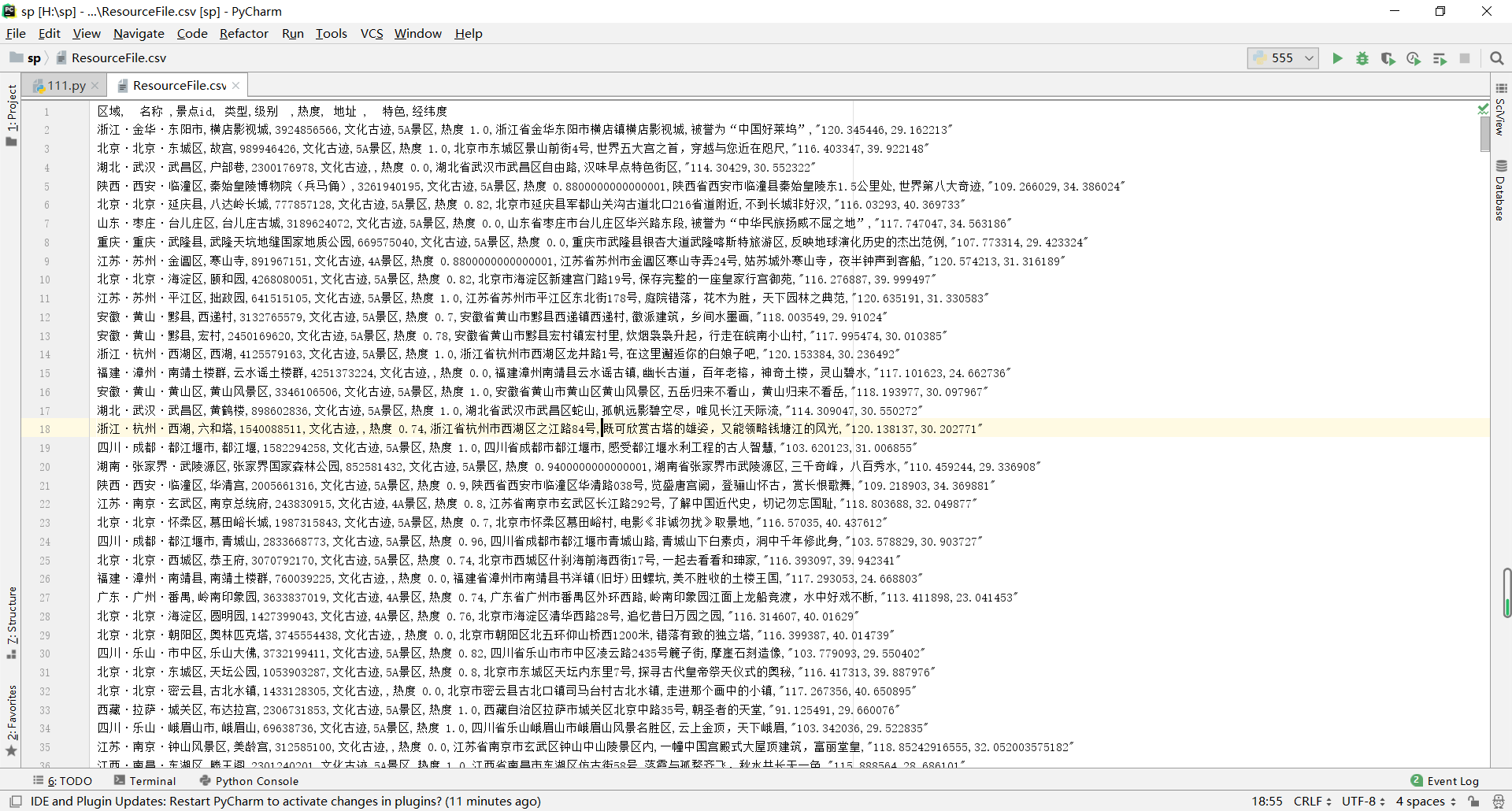数据中主要存在的问题包括：

1.列名中存在空格
2.存在重复数据
3.存在缺失数据

导入pandas模块，打开数据文件

import pandas as pd



当我们想要输出指定列名的时候，却报错了

print(df.名称)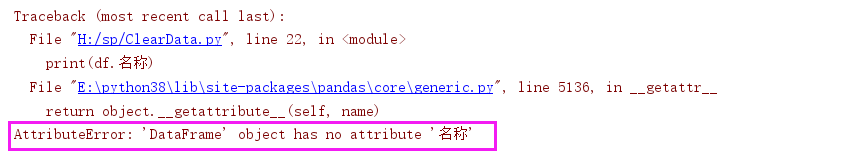但我们的列名中的确是有"名称"这一列的，为什么会显示没有"名称"这一列呢？我们输出列名看一下

方法一：

print(df.describe())方法二：

# 只输出列名
print(df.columns.values)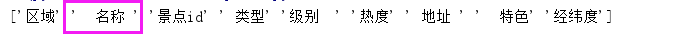我们可以看到，列名"名称"是带有空格的，那如果我们这样写

print(df['  名称 '])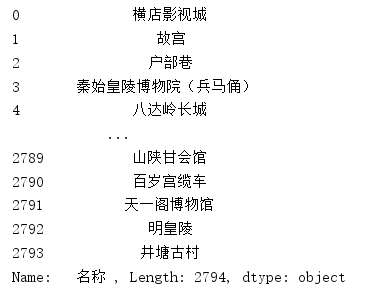就可以输出这一列的数据了

## 所以我们现在要解决的问题就是删除列名中的空格

使用比较简单的一种方法：列表推导式去空格

ClName = df.columns.values
# 使用列表推导式
df.columns = [x.strip() for x in ClName]
print(df.columns.values)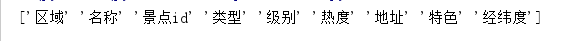成功删除空格

## 接下来要解决的问题就是处理文件中的重复数据

我们先来查看下一共有多少行数据，三种方法

方法一：

# 结果  (行数,列数)
print(df.shape)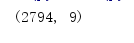方法二：

print(df.info())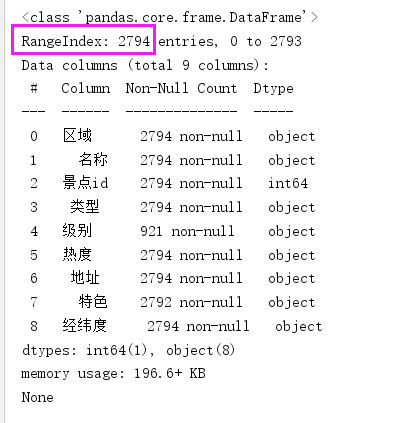方法三：

# 查看索引信息
print(df.index)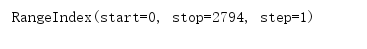我们可以看到，通过三种方法得到的结果是一共有2794行数据

那我们现在来查看重复的数据

这里说的重复是指两行数据完全相同，如果只有部分数据相同，那不是重复

# 判断重复值 返回值类型为 Boolean
print(df.duplicated())


false表示没有重复，true表示有重复，默认从上到下比较，若上一行的数据和下一行的数据重复，则下一行标记为true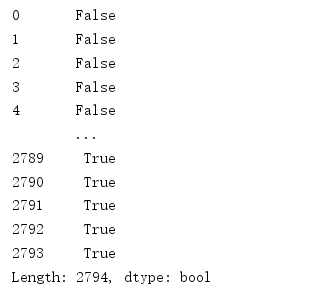如果这样不太直观，我们可以直接查看有多少行重复的数据

# 返回重复的行数
print(df.duplicated().sum())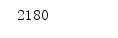还可以查看重复的数据行

# 布尔索引 显示重复数据
print(df[df.duplicated()])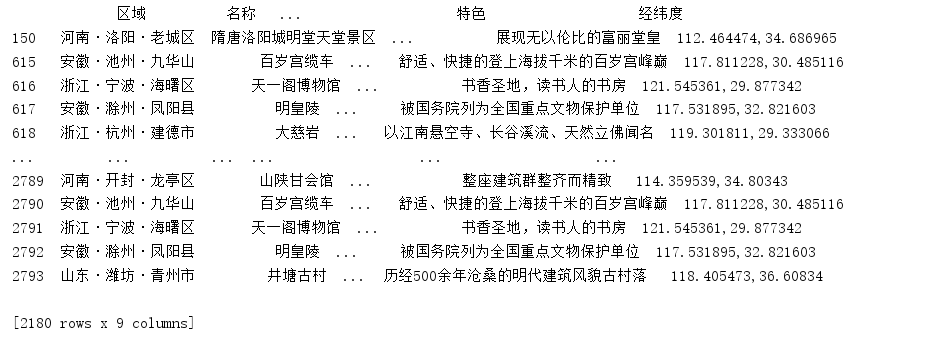接下来就要删除这些重复的数据，两种方法

方法一：

这种方法不会对数据文件直接进行修改，而是生成一个临时表

# 删除重复值 不改变源数据 临时生成的表
print(df.drop_duplicates())


输出的临时表是删除重复数据之后的表，我们可以看到，现在的数据只有614行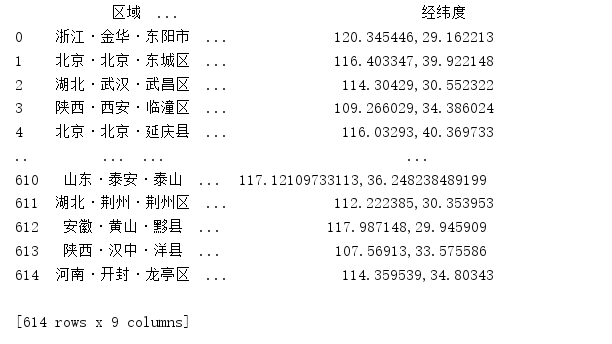而此时如果再查看源数据文件的信息，可以看到，源数据文件中的数据并没有减少

print(df.info())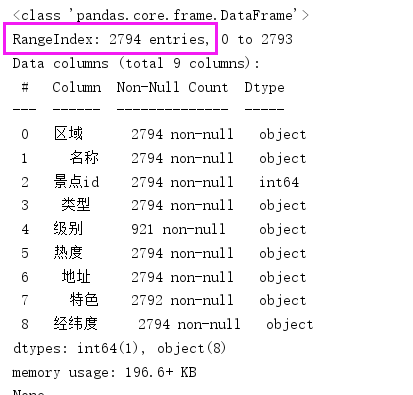方法二：

这种方法会直接对源数据文件进行修改

# 删除重复值 修改源数据
df.drop_duplicates(inplace=True)


查看源数据文件信息

print(df.info())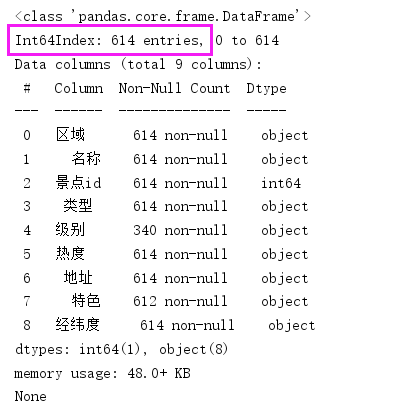删除重复数据之后，我们要重置文件的索引

# 重置索引
df.index = range(df.shape)


查看索引信息

print(df.index)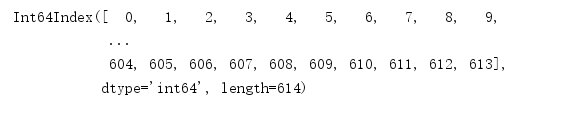## 接下来要处理的问题就是补全数据中的缺失值

第一步，查看缺失值

方法一：

# 查看缺失值
print(df.isnull())


没有缺失值标记为false，有缺失值标记为true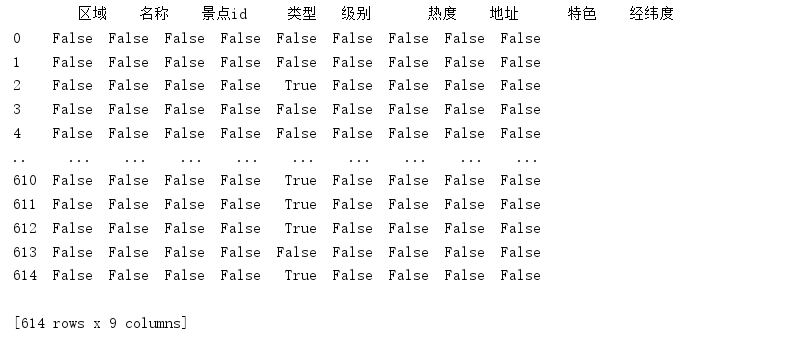方法二：

# 查看没有缺失值
print(df.notnull())


没有缺失值标记为true，有缺失值标记为false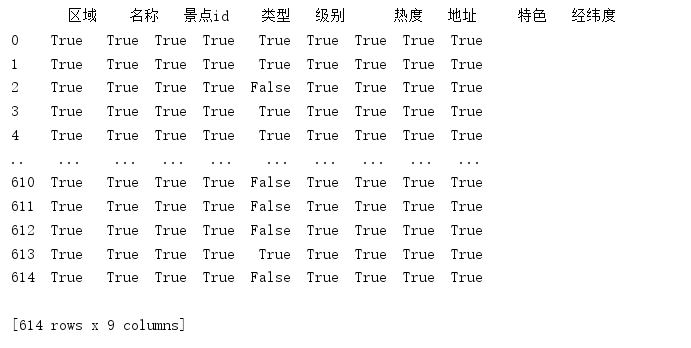方法三：

显示每一列中的缺失值数量

# 显示每一列中的缺失值数量
print(df.isnull().sum())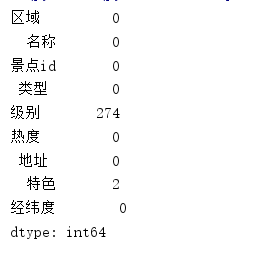方法四：

显示有缺失值的数据

# 显示'特色'列中有缺失值的数据
print(df[df.特色.isnull()])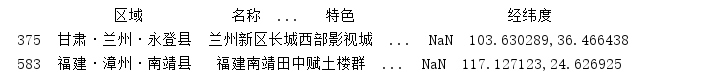第二步，填补缺失值

# 提取'特色'列有缺失值的数据的'区域'列的值
print(df.loc[df.特色.isnull(),'区域'])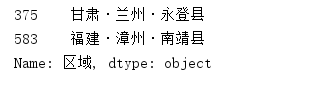填补"级别"列有缺失值的数据，填补内容为"无级别"

# 填补"级别"列有缺失值的数据，填补内容为"无级别"
df.loc[df.级别.isnull(),'级别'] = "无级别"


查看’级别’列中缺失值的数量

print(df.isnull().sum())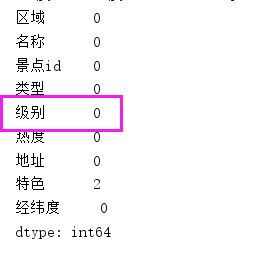填补"特色"列有缺失值的数据，填补内容为"未知"

# 填补"特色"列有缺失值的数据，填补内容为"未知"
df.loc[df.特色.isnull(),'特色'] = "未知"


查看’特色’列中缺失值的数量

print(df.isnull().sum())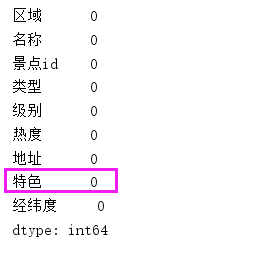数据处理完成，保存文件

df.to_csv("ResourceFile.csv")


查看数据文件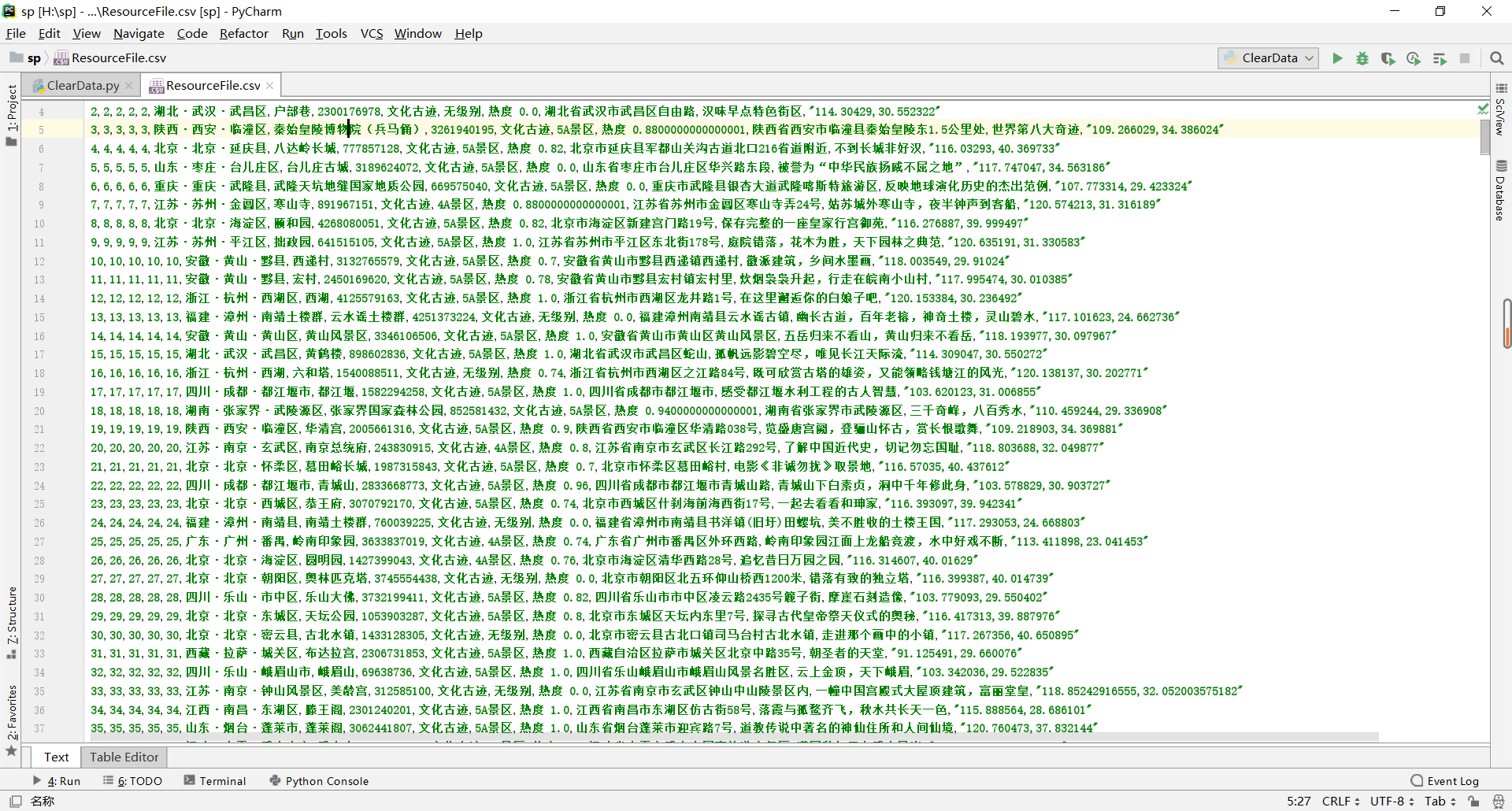## 全部代码

import pandas as pd

# 列名列表
ClName = df.columns.values
# 使用列表推导式
# 列名去空格
df.columns = [x.strip() for x in ClName]
# 删除重复值 修改源数据
df.drop_duplicates(inplace=True)
# 重置索引
df.index = range(df.shape)
# 填补"级别"列有缺失值的数据，填补内容为"无级别"
df.loc[df.级别.isnull(),'级别'] = "无级别"
# 填补"特色"列有缺失值的数据，填补内容为"未知"
df.loc[df.特色.isnull(),'特色'] = "未知"
# 保存文件
df.to_csv("ResourceFile.csv")


展开全文python 数据清洗
• 数据处理、csv、pandas、数据匹配、虚拟变量、分组统计

# 写在前面

本人python新手一枚，最近毕业论文需要用python处理数据，大概就是两年前学过一些python基础，最近重新把python捡起来了，由于这个数据处理过程对我这种新手来说过于艰难，所以想要把它记录下来，方便以后需要的时候再回顾，也希望其他同学遇到类似问题的时候可以更快找到解决方法~

下面正文开始

## 1. 要用到的库

import os
import pandas as pd
import openpyxl
import csv
import xlrd


## 2. 遍历文件夹，获取文件夹下的文件路径

#遍历某个文件夹下的所有文件，并将其路径存储到列表里
#这里只遍历了两层，如果有更多层文件夹的话可以继续往下遍历
def get_files():
l = []
directory = '文件夹路径'
for f in os.listdir(directory):
file_path = os.path.join(directory,f) #第一层
for folder in os.listdir(file_path):
files = os.path.join(file_path, folder) #第二层
l.append(files)
return l
path = get_files()


## 3. 文件合并

for i in path:
df = df.loc[:, ['区域','板块','租金', '被叫手机号', '被叫姓名','被叫时长(秒)', '拨打时间']] #这里定义的是选取该文件的哪些列，全部都保留的话可以省略这条
df.to_csv('文件路径.csv',mode='a',index=False) #这里的'a'是追加的意思，方便合并
print(i)



## 4. 数据清洗

#去除重复数据
df2.drop_duplicates(inplace=True)
print('finish part2')

#把以下列的所有空值赋值为null后，删除含有null的行
df2['被叫手机号'] = df2['被叫手机号'].fillna('null')
df2 = df2[~df2['被叫手机号'].isin(['null'])]#该方法也可以用来删除包含特定字段的行
df2.to_csv('文件路径',mode='w',index=False) #做完去重和缺失值处理后写入文件
print('finish part3')


## 5. 遍历csv文件的每一行

因为我的数据需要，pandas里面我不知道怎么去遍历每一行，所以我用了csv这个包，如果某一行的数据不符合要求就删掉，把符合要求的写进一个新的csv里面

import csv
l = []
f = open('文件路径','r',encoding='utf-8')
f1 = open('文件路径','w+',encoding='utf-8',newline='')
writer = csv.writer(f1)
header = ['区域','板块','租金', '被叫手机号', '被叫姓名','被叫时长(秒)', '拨打时间']
#下面是判断手机号是不是以1开头，长度为11位，如果不是就删掉
#名字里有没有测试这两个字，如果有也删掉
for line in reader:
if line != '1':
#print(line)
continue
if len(line) != 11:
#print(line)
continue
if '测试' in line:
continue
else:
writer.writerow(line)
print('finish')
f.close()
f1.close()



数据清洗阶段基本上就完成了，如果还有其他需求的小伙伴也可以根据上面的内容自行修改。

接下来就是进行一些数据的计算、分组统计和匹配等等，这里仅列出比较通用的部分。

## 6. 数据计算和匹配

### - 分组统计

如果你的数据里同一个id或者同一个时间有多个值，那么就可以用pandas里的groupby函数进行分组计数、求和、求平均值等等。groupby用法很多，可自行百度。

df = pd.read_csv('文件路径',index_col=False)
g = df.groupby(['id','月份'])['被叫次数'].sum().reset_index()#这里是求每个人每个月一个被呼叫了多少次，用的是sum函数。
g.to_csv('文件路径',mode='w',index=False)


如果需要计数的话需要用count()或size()函数，这两个函数的区别是count()只计算有值的数，size则是就算该行有缺失值也会计算进去。

### - 数据匹配

当有两个或多个表格，它们中包含同一个唯一字段（即数据库里的主码）需要根据该字段进行匹配时，可以用pandas的merge函数。

left = pd.read_csv('文件路径',index_col=False)
#right = right.loc[:,['id','房东电话','月份']] 可以只选取其中部分字段
result = pd.merge(left,right,left_on=['注册时间','时间'],right_on=['注册时间','时间'],how="left") #merge用法自行百度, on参数和how参数很重要
result.to_csv('文件路径',mode='w',index=False)
print('finish!')


### - 计算两个时间之间的间隔天数

这个是参考某位大佬的，具体出处戳这里

import time

def demo(day1, day2):
time_array1 = time.strptime(day1, "%Y/%m/%d")#如果分隔符不一样改掉即可
timestamp_day1 = int(time.mktime(time_array1))
time_array2 = time.strptime(day2, "%Y/%m/%d")
timestamp_day2 = int(time.mktime(time_array2))
result = (timestamp_day2 - timestamp_day1) // 60 // 60 // 24
return result


### - 生成虚拟变量

df = pd.read_csv('文件路径',index_col=False)
renttype_dummy = pd.get_dummies(df['租赁类型'])
df = df.join(renttype_dummy)
df.to_csv('文件路径',mode='w',index=False)


### - 判断某个房子在某月是否处于上架状态

（在上架日期和下架日期之间）
这其实是一个非常笨的办法，但我也想不到其他的了…
逻辑就是把上架日期和下架日期的年份和月份取出来合并到一起，即201701这种形式，然后比较大小即可。
例如201709 < 201710 <201801，那这个房子在201710的时候肯定是处于上架状态的。

#计算房东每月总房源数量
f = open('文件路径','r',encoding='utf-8')
f1 = open('文件路径','w',encoding='utf-8')
writer = csv.writer(f1)
for line in reader:
xiajia = line[-6].split('/')#用分隔符把年月日分开
print(xiajia)
shangjia = line[-5].split('/')#用分隔符把年月日分开
if len(xiajia) == 1:
month1 = '0' + xiajia#以/为分隔符的日期一般月份都是不带0的
xjdate = xiajia + month1
else:
xjdate = xiajia + xiajia
if len(shangjia) == 1:
month2 = '0' + shangjia
sjdate = shangjia + month2
#print(sjdate)
else:
sjdate = shangjia + shangjia
#print(sjdate)
#下面就是判断它属于哪个月，有可能同时属于好几个月
if int(sjdate) <= 201701 <= int(xjdate):
l = []
l.append(line)
l.append(line)
l.append('201701')
writer.writerow(l)
if int(sjdate) <= 201702 <= int(xjdate):
l = []
l.append(line)
l.append(line)
l.append('201702')
writer.writerow(l)
if int(sjdate) <= 201703 <= int(xjdate):
l = []
l.append(line)
l.append(line)
l.append('201703')
writer.writerow(l)
if int(sjdate) <= 201704 <= int(xjdate):
l = []
l.append(line)
l.append(line)
l.append('201704')
writer.writerow(l)
if int(sjdate) <= 201705 <= int(xjdate):
l = []
l.append(line)
l.append(line)
l.append('201705')
writer.writerow(l)
if int(sjdate) <= 201706 <= int(xjdate):
l = []
l.append(line)
l.append(line)
l.append('201706')
writer.writerow(l)
if int(sjdate) <= 201707 <= int(xjdate):
l = []
l.append(line)
l.append(line)
l.append('201707')
writer.writerow(l)
if int(sjdate) <= 201708 <= int(xjdate):
l = []
l.append(line)
l.append(line)
l.append('201708')
writer.writerow(l)
if int(sjdate) <= 201709 <= int(xjdate):
l = []
l.append(line)
l.append(line)
l.append('201709')
writer.writerow(l)
if int(sjdate) <= 201710 <= int(xjdate):
l = []
l.append(line)
l.append(line)
l.append('201710')
writer.writerow(l)
if int(sjdate) <= 201711 <= int(xjdate):
l = []
l.append(line)
l.append(line)
l.append('201711')
writer.writerow(l)
if int(sjdate) <= 201712 <= int(xjdate):
l = []
l.append(line)
l.append(line)
l.append('201712')
writer.writerow(l)
if int(sjdate) <= 201801 <= int(xjdate):
l = []
l.append(line)
l.append(line)
l.append('201801')
writer.writerow(l)
if int(sjdate) <= 201802 <= int(xjdate):
l = []
l.append(line)
l.append(line)
l.append('201802')
writer.writerow(l)
if int(sjdate) <= 201803 <= int(xjdate):
l = []
l.append(line)
l.append(line)
l.append('201803')
writer.writerow(l)
if int(sjdate) <= 201804 <= int(xjdate):
l = []
l.append(line)
l.append(line)
l.append('201804')
writer.writerow(l)
if int(sjdate) <= 201805 <= int(xjdate):
l = []
l.append(line)
l.append(line)
l.append('201805')
writer.writerow(l)
if int(sjdate) <= 201806 <= int(xjdate):
l = []
l.append(line)
l.append(line)
l.append('201806')
writer.writerow(l)
if int(sjdate) <= 201807 <= int(xjdate):
l = []
l.append(line)
l.append(line)
l.append('201807')
writer.writerow(l)
if int(sjdate) <= 201808 <= int(xjdate):
l = []
l.append(line)
l.append(line)
l.append('201808')
writer.writerow(l)
if int(sjdate) <= 201809 <= int(xjdate):
l = []
l.append(line)
l.append(line)
l.append('201809')
writer.writerow(l)
if int(sjdate) <= 201810 <= int(xjdate):
l = []
l.append(line)
l.append(line)
l.append('201810')
writer.writerow(l)
if int(sjdate) <= 201811 <= int(xjdate):
l = []
l.append(line)
l.append(line)
l.append('201811')
writer.writerow(l)
if int(sjdate) <= 201812 <= int(xjdate):
l = []
l.append(line)
l.append(line)
l.append('201812')
writer.writerow(l)
if int(sjdate) <= 201901 <= int(xjdate):
l = []
l.append(line)
l.append(line)
l.append('201901')
writer.writerow(l)
if int(sjdate) <= 201902 <= int(xjdate):
l = []
l.append(line)
l.append(line)
l.append('201902')
writer.writerow(l)
if int(sjdate) <= 201903 <= int(xjdate):
l = []
l.append(line)
l.append(line)
l.append('201903')
writer.writerow(l)
if int(sjdate) <= 201904 <= int(xjdate):
l = []
l.append(line)
l.append(line)
l.append('201904')
writer.writerow(l)
if int(sjdate) <= 201905 <= int(xjdate):
l = []
l.append(line)
l.append(line)
l.append('201905')
writer.writerow(l)
if int(sjdate) <= 201906 <= int(xjdate):
l = []
l.append(line)
l.append(line)
l.append('201906')
writer.writerow(l)
if int(sjdate) <= 201907 <= int(xjdate):
l = []
l.append(line)
l.append(line)
l.append('201907')
writer.writerow(l)
if int(sjdate) <= 201908 <= int(xjdate):
l = []
l.append(line)
l.append(line)
l.append('201908')
writer.writerow(l)
if int(sjdate) <= 201909 <= int(xjdate):
l = []
l.append(line)
l.append(line)
l.append('201909')
writer.writerow(l)
if int(sjdate) <= 201910 <= int(xjdate):
l = []
l.append(line)
l.append(line)
l.append('201910')
writer.writerow(l)
if int(sjdate) <= 201911 <= int(xjdate):
l = []
l.append(line)
l.append(line)
l.append('201911')
writer.writerow(l)
if int(sjdate) <= 201912 <= int(xjdate):
l = []
l.append(line)
l.append(line)
l.append('201912')
writer.writerow(l)
f.close()
f1.close()


最最后，立个小小的flag，今年一定要更深入的学习python和sql，希望所有的数据人都能快乐（哭）。
如果有大佬有更好的方法，请多多指教。

展开全文python
• 2、看数据大致情况 data.info() 3、当表很大的时候想看表长什么样子 data.head(n)#显示前n行 4、删除表的若干列 data.trop(['aaa','bbb','ccc'],inplace = True,axis = 1)#删除表头为aaa,bbb,ccc的列，inplace = ...

1、读取CSV文件

data = pd.read_csv(r'.\')


2、看数据大致情况

data.info()


3、当表很大的时候想看表长什么样子

data.head(n)#显示前n行


4、删除表的若干列

data.trop(['aaa','bbb','ccc'],inplace = True,axis = 1)#删除表头为aaa,bbb,ccc的列，inplace = True并用后来的表去覆盖前面的表


5、用均值填补缺失值

data['age'] = data['age'].fillna(data['age'].mean())


6、删掉有缺失值的行

data = data.drpona()#默认axis = 0


7、看一列表头里面到底有多少类别

data['ddd'].unique()


8、讲上面的类别[‘s’,‘q’,‘k’]转化为[0,1,2]供分类

labels = data['Embarked'].unique().tolist()
data['Embarked'] = data['Embarked'].apply(lambda x: labels.index(x))


9、把性别转换为0，1

data['Sex'] = (data['Sex'] == 'male').astype('int')


10、取出除了某一列外的数据

x = data.iloc[:,data.columns != 'kkk']


11、乱序的索引按顺序排列

xtrain.index = range(xtrain.shape)


12、训练并交叉验证

clf = DecisionTreeClassifier(random_state = 25)
score = cross_val_score(clf,x,y,cv=10).mean()


13、网格搜索

import numpy as np
gini_thresholds = np.linspace(0,0.5,20)
parameters = {'splitter':('best','random')
,'criterion':("gini","entropy")
,"max_depth":[*range(1,10)]
,'min_samples_leaf':[*range(1,50,5)]
,'min_impurity_decrease':[*np.linspace(0,0.5,20)]
}
clf = DecisionTreeClassifier(random_state=25)
GS = GridSearchCV(clf, parameters, cv=10)
GS.fit(Xtrain,Ytrain)
GS.best_params_
GS.best_score_

展开全文python 数据挖掘
• 本次课程主要以真实的电商数据为基础，通过Python详细的介绍了数据分析中的数据清洗阶段各种技巧和方法。编程语言 Python python
• 主要介绍了Python123 CSV格式清洗与转换的实例代码，非常不错，具有一定的参考借鉴价值,需要的朋友可以参考下
• 本次课程主要以真实的电商数据为基础，通过Python详细的介绍了数据分析中的数据清洗阶段各种技巧和方法。编程语言 Python python
• import csv class WriteCSV(): """定义成员变量""" def __init__(self): pass def init(self, info_list, info_dict_list): self.info_list = info_list self.info_dict_list = info_dict_list def ...
• 提取CSV格式文件数据进行如下格式转换：‪‬‪‬‪‬‪‬‪‬‮‬‫‬‪‬‪‬‪‬‪‬‪‬‪‬‮‬‫‬‭‬‪‬‪‬‪‬‪‬‪‬‮‬‫‬‭‬‪‬‪‬‪‬‪‬‪‬‮‬‪‬‪‬‪‬‪‬‪‬‪‬‪‬‮‬‫‬‭‬‪...python
• 无论是做机器学习还是做数据分析，都离不开获取数据后的第一步-数据清洗工作。据统计，数据清洗工作占据整个工作时间百分之50左右，有的甚至能达到百分之70。下面我将介绍我进行数据清洗得思路流程。 数据清洗整体...
• python数据清洗与准备：缺失值、重复值、异常值处理python 机器学习
• ·pandas内置了10多种数据源读取函数，常见的就是CSV和Excel ·使用read_csv方法读取，结果为dataframe格式 ·在读取CSV文件时，文件名尽量是英文 ·参数较多，可以自行控制，但很多时候用默认参数 ·在读取CSV时，...python
• csv文件读写 pandas内置了10多种数据源读取函数，常见的就是CSV和EXCEL 使用read_csv方式读取。结果为dataframe格式 ...'D:\\Jupyter\\notebook\\Python数据清洗实战\\数据清洗之文件读写' os.chdir('D
• 本次课程主要以真实的电商数据为基础，通过Python详细的介绍了数据分析中的数据清洗阶段各种技巧和方法。编程语言 Python python
• Python数据清洗csv reader zip融合 需求概述 借助Python的CSV通过reader方法实现便利，并通过循环匹配在另外个csv里找到当前CSV字段的中文注释，将都能相互匹配到的列（字段）结合数据通过zip拼接后输出来。 ...python
• 附件是一个CSV文件，其中每个数据前后存在空格，请对其进行清洗，要求如下：‪‬‪‬‪‬‪‬‪‬‮‬‪‬‭‬‪‬‪‬‪‬‪‬‪‬‮‬‫‬‭‬‪‬‪‬‪‬‪‬‪‬‮‬‪‬‫‬‪‬‪‬‪‬‪‬‪‬‮‬‫‬‭‬...python
• 文章目录数据清洗步骤函数大全数据清洗的内容总结 数据清洗步骤 数据获取，使用read_csv或者read_excel 数据探索，使用shape，describe或者info函数 行列操作，使用loc或者iloc函数 数据整合，对不同的数据源进行...
• 提取码：23uk数据清洗意义数据清洗常用工具数据库建立连接参数 conn =create_engine(‘mysql+pymysql://user:passward@IP:3306/test01’) root: 用户名 passward: 密码 IP : 服务器IP，本地电脑用localhost 3306： ...python 数据挖掘
• 目录python数据清洗学习记录–文件读写csv文件读写excel文件读写数据库文件的读写创建连接，连接MySQL数据保存 前期准备： #导入os模块 import os #获得当前路径地址 os.getcwd() # 更改文件路劲 os.chdir('F:\...
• 数据清洗，使用python数据清洗cvs里面带中文字符，意图是用字典对应中文字符，即key值是中文字符，value值是index，自增即可；利用字典数据结构没有重复key值的特性，把中文字符映射到了数值index。python代码如下：...
• 本次课程主要以真实的电商数据为基础，通过Python详细的介绍了数据分析中的数据清洗阶段各种技巧和方法。编程语言 Python python
• 附件是一个 CSV 文件，其中每个数据前后存在空格，请对其进行清洗，要求如下：‪‬‪‬‪‬‪‬‪‬‮‬‭‬‫‬‪‬‪‬‪‬‪‬‪‬‮‬‪‬‫‬‪‬‪‬‪‬‪‬‪‬‮‬‪‬‮‬‪‬‪‬‪‬‪‬‪‬‮‬‫‬‪...python
• ## Python数据清洗——Pandas

千次阅读 多人点赞 2021-07-22 19:16:54
简单理解为以右表内容为主，进行查询，右表有，左表没有的内容会被以NaN填满 2.8 数据保存与读取 2.8.1 保存 数据保存，把在python中操作后的表格存到本地 1、df有被定义 2、df.to_excel() or df.to_csv() 3、可以...python 数据清洗 数据挖掘 jupyter...